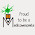## Wednesday, December 3, 2014

### Total iron dose infusion formula (Haldane and Ganzoni) mnemonic

Hi everyone!
We'll be learning about an iron and anemia related topic today.

When a patient has severe anemia, you need to calculate the TDI (Total dose infusion) for parenteral therapy.

And there are various formulas with all sorts of numbers in em.
I'm allergic to memorization but I love memory aids! ;)

Haldane formula for TDI = 0.3 x weight (lbs) x (100 -  Hb %) + 50%

Haldane's formula mnemonic:
H has 3 strokes | - |, so 0.3 is the multiplication factor.
Haldane has a L, for weight in Lbs.
Haldane has a H, H for Hundred. So hundred minus Hb %.
Add half of hundred, 50%, for stores.

Ganzoni formula for TDI = 2.4 x weight (kg) x (15 - Hb %) + 500 mg

Ganzoni formula mnemonic:
(You need to be thorough with Haldane's formula for this one, because it's a comparison with Haldane's formula, finding analogies and differences.)
Haldane had 3, so 2 and 4 come in Ganzoni's formula as a multiplication factor.
Ganzoni has a G for weight in kiloGram, storage dose in milliGram. (Instead of % in Haldane's!)
GanzOni has an extra O, for addition of 500 instead of 50 from Haldane's.

Other random iron related things I remember -
That's how I remember iron sorbitol isn't given intravenously.

"Glue sulphur fumes"
That's how I remember concentrations of iron preparations, from low to high.
Ferrous gluconate is given 12%
Ferrous sulphate 30%
Ferrous fumarate has 2 f's so 33%

That's all!
I admit that it's extremely stupid. (But you guys already know that that's how I roll!)

1.Haha , i like your tricky ways , good work

1.Thank you Raman! :D

This is express yourself space. Where you type create something beautiful! <3
Wondering what do I write? Well...
Tell us something you know better. You are a brilliant mind. Yes, you are! ^__^